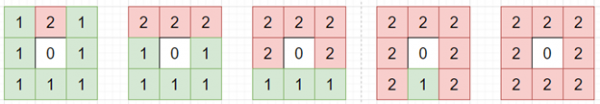# Program to find number of steps to solve 8-puzzle in python

PythonServer Side ProgrammingProgramming

Suppose we have a 3x3 board of where all numbers are in range 0 to 8 and no repeating numbers are there. Now, we can swap the 0 with one of its 4 neighbors, and we are trying to solve it to get all arranged sequence, we have to find minimum number of steps required to reach the goal.

So, if the input is like

 3 1 2 4 7 5 6 8 0

then the output will be 4To solve this, we will follow these steps −

• Define a function find_next() . This will take node
• moves := a map defining moves as a list corresponding to each value {0: [1, 3],1: [0, 2, 4],2: [1, 5],3: [0, 4, 6],4: [1, 3, 5, 7],5: [2, 4, 8],6: [3, 7],7: [4, 6, 8],8: [5, 7],}
• results := a new list
• pos_0 := first value of node
• for each move in moves[pos_0], do
• new_node := a new list from node
• swap new_node[move] and new_node[pos_0]
• insert a new tuple from new_node at the end of results
• return results
• Define a function get_paths() . This will take dict
• cnt := 0
• Do the following infinitely, do
• current_nodes := a list where value is same as cnt
• if size of current_nodes is same as 0, then
• return -1
• for each node in current_nodes, do
• next_moves := find_next(node)
• for each move in next_moves, do
• if move is not present in dict, then
• dict[move] := cnt + 1
• if move is same as (0, 1, 2, 3, 4, 5, 6, 7, 8) , then
• return cnt + 1
• cnt := cnt + 1
• From the main method do the following:
• dict := a new map, flatten := a new list
• for i in range 0 to row count of board, do
• flatten := flatten + board[i]
• flatten := a copy of flatten
• dict[flatten] := 0
• if flatten is same as (0, 1, 2, 3, 4, 5, 6, 7, 8) , then
• return 0
• return get_paths(dict)

Let us see the following implementation to get better understanding −

## Example

Live Demo

class Solution:
def solve(self, board):
dict = {}
flatten = []
for i in range(len(board)):
flatten += board[i]
flatten = tuple(flatten)

dict[flatten] = 0

if flatten == (0, 1, 2, 3, 4, 5, 6, 7, 8):
return 0

return self.get_paths(dict)

def get_paths(self, dict):
cnt = 0
while True:
current_nodes = [x for x in dict if dict[x] == cnt]
if len(current_nodes) == 0:
return -1

for node in current_nodes:
next_moves = self.find_next(node)
for move in next_moves:
if move not in dict:
dict[move] = cnt + 1
if move == (0, 1, 2, 3, 4, 5, 6, 7, 8):
return cnt + 1
cnt += 1

def find_next(self, node):
moves = {
0: [1, 3],
1: [0, 2, 4],
2: [1, 5],
3: [0, 4, 6],
4: [1, 3, 5, 7],
5: [2, 4, 8],
6: [3, 7],
7: [4, 6, 8],
8: [5, 7],
}

results = []
pos_0 = node.index(0)
for move in moves[pos_0]:
new_node = list(node)
new_node[move], new_node[pos_0] = new_node[pos_0], new_node[move]
results.append(tuple(new_node))

return results
ob = Solution()
matrix = [
[3, 1, 2],
[4, 7, 5],
[6, 8, 0]
]
print(ob.solve(matrix))

## Input

matrix = [
[3, 1, 2],
[4, 7, 5],
[6, 8, 0] ]

## Output

4